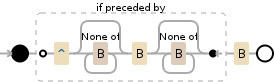Replace the second char of a string

I have a string variable.

Dim str As String = "ABBCD"

I want to replace only the second 'B' character of str (I mean the second occurrence)

my code

Dim regex As New Regex("B")
Dim result As String = regex.Replace(str, "x", 2)

'result: AxxCD
'but I want: ABxCD

What's the easiest way to do this with Regular Expressions.

thanks

Dim str As String = "ABBCD"
Dim matches As MatchCollection = Regex.Matches(str, "B")
If matches.Count >= 2 Then
str = str.Remove(matches(1).Index, matches(1).Length)
str = str.Insert(matches(1).Index, "x")
End If

First we declare the string 'str', then find the matches of "B". If we found two results or more, replace the second result with "x".

• Please add some commentary to your answers to explain why this code solves the problem. Code-only answers are often not very useful for beginners. – ebarr May 4 '14 at 22:38
• Okay, first we declare the string 'str' Then find the matches of "B" If we found two results or more, replace the second result with "x" – Aly El-Haddad May 4 '14 at 22:55
• Edit your answer with the commentary. – ebarr May 4 '14 at 23:27
• that's what I really want to do. I just want to replace only the second "B" (not, after second or last "B") and also can adjustable such as third "B" or fourth etc. thank you. – samgi May 5 '14 at 3:27

resultString = Regex.Replace(subjectString, @"(B)\1", "\$+x");
• It's also Works. thank you. – samgi May 4 '14 at 19:31
• Also try to post an explanation – Rich May 4 '14 at 19:48

Use a positive lookbehind:

Dim regex As New Regex("(?<=B)B")

Live demo

• I'm sorry I cant vote up answers yet. thank you – samgi May 4 '14 at 19:33
• @samgi You're welcome instead you can "accept" one. – revo May 4 '14 at 19:36

If ABCABCABC should produce ABCAxCABC, then the following regex will work:

(?<=^[^B]*B[^B]*)BUsage:

Dim result As String = Regex.Replace(str, "(?<=^[^B]*B[^B]*)B", "x")
• very useful answer thank you. – samgi May 5 '14 at 3:28

I assume BB was just an example, it can be CC, DD, EE, etc..
Based on that, the regex below will replace any repeated character in the string.

resultString = Regex.Replace(subjectString, @"(\w)\1", "\$1x");
'Alternative way to replace the second occurrence
'only of B in the string with X
Dim str As String = "ABBCD"
Dim pattern As String = "B"
Dim reg As Regex = New Regex(pattern)
Dim replacement As String = "X"
'find position of second B
Dim secondBpos As Integer = Regex.Matches(str, pattern)(1).Index
'replace that B with X
Dim result As String = reg.Replace(str, replacement, 1, secondBpos)
MessageBox.Show(result)